# Comparing and Sorting in Java

#### View more Tutorials:## 1- Primitive type and reference type in Java

First of all, we need to differentiate the Primitive type and the Reference type in Java.
In Java we have 8 primitives.
• Primitive type
Type Bit/Bytes Range
boolean 1 bit True or False
char 16 bit/ 2 bytes 0 to 65535
byte 8 bit/ 1 byte -128 to 127
short 16 bit/ 2 bytes -32768 to 32767
int 32 bits/ 4 bytes -2147483648 to 2147483647
long 64 bits/ 8 bytes -9,223,372,036,854,775,808 to -9,223,372,036,854,775,808
(-2^63 to -2^63)
float 32 bits/ 4 bytes -3.4028235 x 10^38 to 3.4028235 x 10^38
double 64 bits/ 8 bytes -1.7976931348623157 x 10^308 to 1.7976931348623157 x 10^308
All other types are extended from Object, they are reference types.

## 2- Where are primitive types stored in memory

First, Java does not really make any guarantees that variables will correspond to memory locations; for example, your method might be optimized in such a way that 'i' is stored in a register — or might not even be stored at all, if the compiler can see that you never actually use its value, or if it can trace through the code and use the appropriate values directly.
See a code snippet:
```// Create a variable 'a', and assin value to it.
int a = 100;

// Assign new value to 'a'
a = 200;

// Create variable b, assign b = a.
int b = a;
```
Java takes the following actions: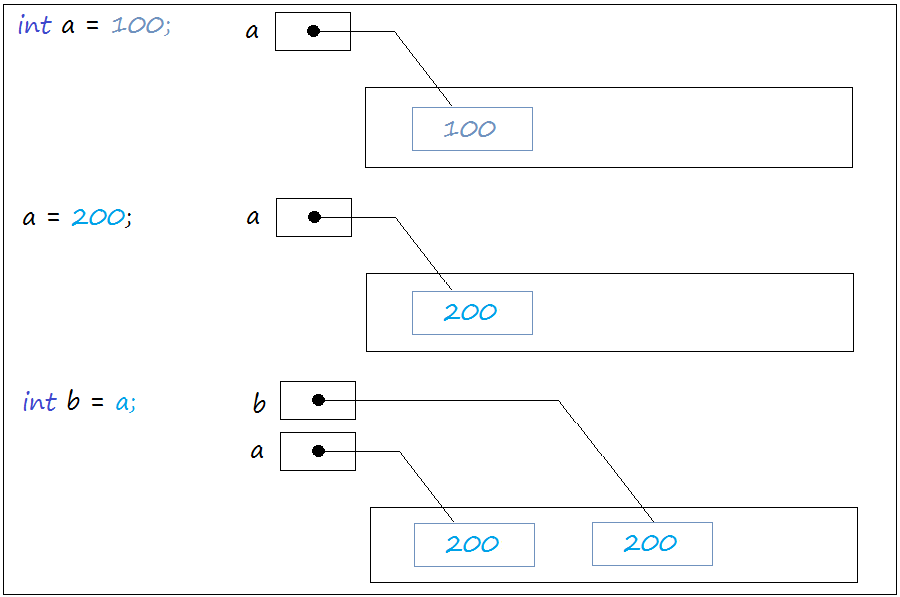• TODO

## 3- Where are reference types stored in memory

When you use the  new operator (for example  new Object ()), Java will create a new entity on memory. You declare a variable and initialize its value through the new operator, such as  Object a = new Object (); Java will create a new entity in the memory, and a reference point to a memory location of the newly created entity.

When you declare a variable b  Object b = a; there is no entity has been created in memory, Java only creates a reference b, point to the same location where a is pointing to.
```// Declare and initialize an object.
Object a =  new Object();

// Assign new value to object 'a'.
a = new String("Text");

// Declare object 'b', and assign 'a' to it.
Object b =  a;
```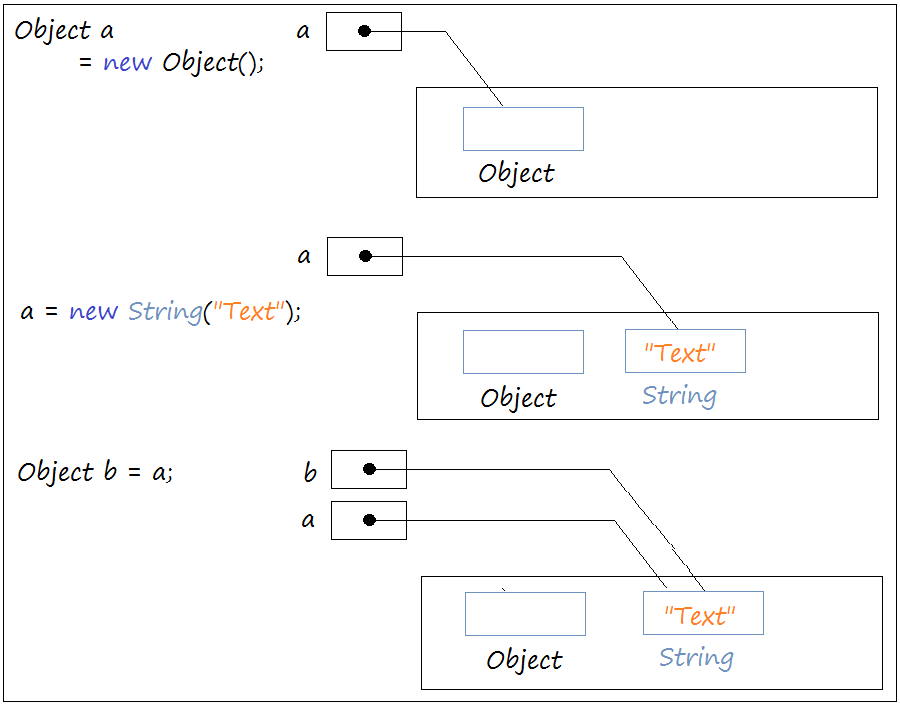• TODO

## 4- The types of comparison in Java

Java has two types of comparisons:
• Use the  operator ==
• Using the  equals(..) method
Operator == used to compare the primitive and reference types.
Equals operator(..) is the method only used for reference types.

## 5- Comparison of primtive types

With primitive type, we have only one way to compare by  using the operator ==
```// Create  variable 'a', assign a value of 200 to it.
// A memory area (1) is created that contains the value of 200.
int a = 200;

// Create a variable 'b', assign a value of 200 to it.
// A memory area (2) is created that contains the value of 200.
int b = 200;

// Although 'a' and 'b' point to two different memory areas.
// Comparing a == b will returns true.
// Because primitive types are compared against each other based on value.
boolean c = (a == b);
```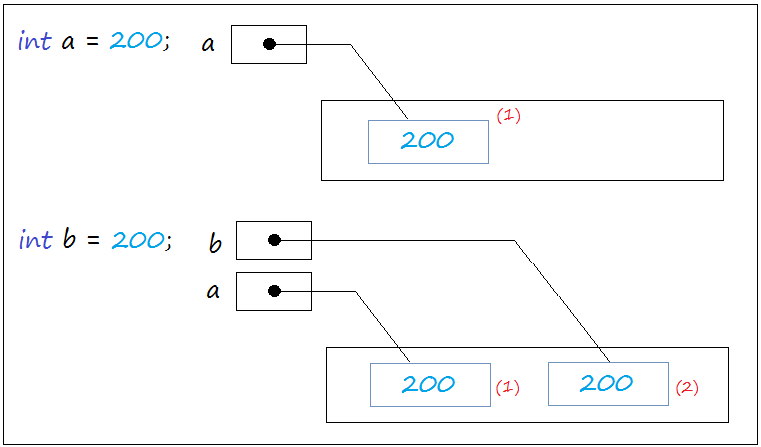## 6- Comparison of reference types

### 6.1- Using the == operator to compare reference types

When you compare two object reference by using the == operator, which means comparing position where 2  object reference are pointing to. Essentially,   it is to check whether 2  object reference   are pointing to the same entity on memory or not.
Consider, for example:
ReferenceEeDemo.java
```package org.o7planning.tutorial.comparation;

public class ReferenceEeDemo {

public static void main(String[] args) {

// NOTE: For String, two ways to initialize the object below are not the same:
String str1 = "String 1";
String str2 = new String("String 1");

// The 'new' operator create the memory area (1)
// Contains the "This is text" String
// And 's1' is a reference that points to (1).
String s1 = new String("This is text");

// The 'new' operator create the memory area (2)
// Contains the "This is text" String
// And s2 is a reference that points to (2)
String s2 = new String("This is text");

// Use the operator== to compare 's1' and 's2'.
// Results is false.
// It is obviously different from what you think.
// The reason is the type of reference
// Operator == compares the positions that they point to.
boolean e1 = (s1 == s2); // false

System.out.println("s1 == s2 ? " + e1);

// Without any 'new' operator.
// Java creates a reference named 'obj'
// And pointing to a memory area that 's1' points to.
Object obj = s1;

// 2 references 'obj' and 's1' are all pointing to one area of memory.
// Results is true.
boolean e2 = (obj == s1); // true

System.out.println("obj == s1 ? " + e2);
}

}
```
Running example: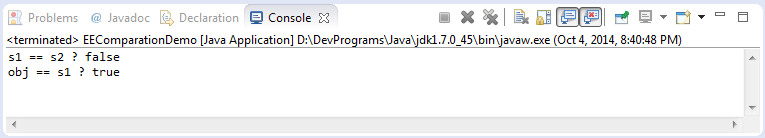### 6.2- Using equals(..) to compare reference types

StringComparationDemo.java
```package org.o7planning.tutorial.comparation;

public class StringComparationDemo {

public static void main(String[] args) {

String s1 = new String("This is text");

String s2 = new String("This is text");

// s1 and s2 Comparison, use equals(..)
boolean e1 = s1.equals(s2);

// The result is true
System.out.println("first comparation: s1 equals s2 ? " + e1);

s2 = new String("New s2 text");

boolean e2 = s1.equals(s2);

// The result is false
System.out.println("second comparation: s1 equals s2 ? " + e2);
}

}
```
Running example: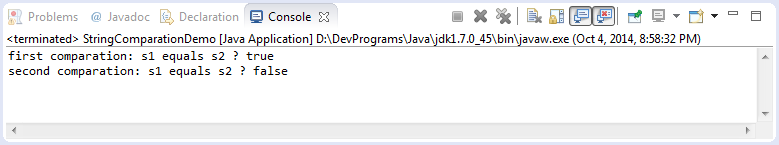### 6.3- Override equals(Object) method

equals(Object) method is a available method of the Object class, all subclasses is inherited this method. In some situations,you can override this method in subclasses.
NumberOfMedals.java
```package org.o7planning.tutorial.comparation.equals;

// Number of medals.
public class NumberOfMedals {

// Number of gold medals
private int goldCount;

// Number of silver medals
private int silverCount;

// Number of bronze medals
private int bronzeCount;

public NumberOfMedals(int goldCount, int silverCount, int bronzeCount) {
this.goldCount = goldCount;
this.silverCount = silverCount;
this.bronzeCount = bronzeCount;
}

public int getGoldCount() {
return goldCount;
}

public int getSilverCount() {
return silverCount;
}

public int getBronzeCount() {
return bronzeCount;
}

// Override the equals(Object) method of Object class.
@Override
public boolean equals(Object other) {
// if other is null, then return false.
if (other == null) {
return false;
}
// If the 'other' is not type of NumberOfMedals
// then return false
if (!(other instanceof NumberOfMedals)) {
return false;
}

NumberOfMedals otherNoM = (NumberOfMedals) other;

if (this.goldCount == otherNoM.goldCount && this.silverCount == otherNoM.silverCount
&& this.bronzeCount == otherNoM.bronzeCount) {
return true;
}
return false;
}

}
```
NumberOfMedalsComparationDemo.java
```package org.o7planning.tutorial.comparation.equals;

public class NumberOfMedalsComparationDemo {

public static void main(String[] args) {

// US team achievement
NumberOfMedals american = new NumberOfMedals(40, 15, 15);

// Japan team achievements
NumberOfMedals japan = new NumberOfMedals(10, 5, 20);

// South Korean team achievements
NumberOfMedals korea = new NumberOfMedals(10, 5, 20);

System.out.println("Medals of American equals Japan ? " + american.equals(japan));

System.out.println("Medals of Korea equals Japan ? " + korea.equals(japan));
}

}
```
Running example: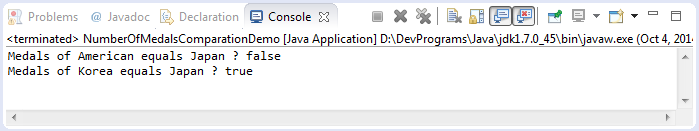## 7- Sorting an array of String example

String is  a class that its objects can be compared with each other, according to the rules of letters. The following example illustrates how to arrange a String array using the  available utility methods in Java.
StringArraySortingDemo.java
```package org.o7planning.tutorial.sorting;

import java.util.Arrays;

public class StringArraySortingDemo {

public static void main(String[] args) {
String[] fruits = new String[] { "Pineapple", "Apple", "Orange", "Banana" };

// Use a static method of Arrays to sort.
// Arrays.sort(Object[])
Arrays.sort(fruits);

for (int i = 0; i < fruits.length; i++) {
System.out.println("fruits " + i + " : " + fruits[i]);
}
}

}
```
Running example: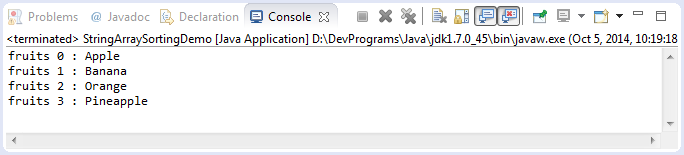## 8- Objects can be compared with each other (Comparable)

```// You write a class which simulates an actor (Actor).
// You want to arrange the order of actors according to the principle of:
// Compare last name (lastName) first, compare first name (firstName).
public class Actor {

// First Name
private String firstName;
// Last Name
private String lastName;

}
```
Actor.java
```package org.o7planning.tutorial.sorting;

// To be compared with each other,
// Actor class must implements Comparable interface.
public class Actor implements Comparable<Actor> {

private String firstName;
private String lastName;

public Actor(String firstName, String lastName) {
this.firstName = firstName;
this.lastName = lastName;
}

public String getFirstName() {
return firstName;
}

public String getLastName() {
return lastName;
}

// Compare this Actor with another Actor.
// According to comparison principle of lastName first,
// then compare firstName.
@Override
public int compareTo(Actor other) {

// Compare 2 String.
int value = this.lastName.compareTo(other.lastName);

// If lastName of two objects are not equals.
if (value != 0) {
return value;
}
// If lastName of 2 objects is the same.
// Next, compare firstName.
value = this.firstName.compareTo(other.firstName);
return value;
}

}
```
ActorSortingDemo.java
```package org.o7planning.tutorial.sorting;

public class ActorSortingDemo {

public static void main(String[] args) {

Actor actor1 = new Actor("Mischa", "Barton");
Actor actor2 = new Actor("Christian", "Bale");
Actor actor3 = new Actor("Joan", "Collins");
Actor actor4 = new Actor("Gemma", "Arterton");
Actor actor5 = new Actor("Daniel", "Craig");

Actor[] actors = new Actor[] { actor1, actor2, actor3, actor4, actor5 };

// Use an algorithm to re-sort the array above.
// Sort the Actor objects in gradually increase order.
for (int i = 0; i < actors.length; i++) {

for (int j = i + 1; j < actors.length; j++) {
// If actors[j] < actors[i]
// Then swap positions with each other.
if (actors[j].compareTo(actors[i]) < 0) {
// Use a temporary variable.
Actor temp = actors[j];
actors[j] = actors[i];
actors[i] = temp;
}
}
}
// Print out elements
for (int i = 0; i < actors.length; i++) {
System.out.println(actors[i].getFirstName() + "  " + actors[i].getLastName());
}

}

}
```
Running example: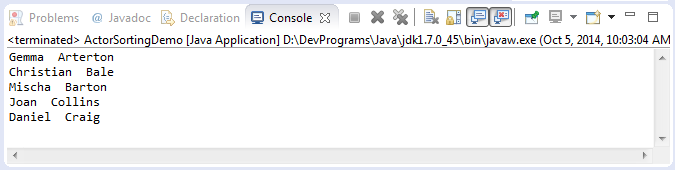Use Arrays.sort(Object []) to sort the list of example above:
ActorSortingDemo2.java
```package org.o7planning.tutorial.sorting;

import java.util.Arrays;

public class ActorSortingDemo2 {

public static void main(String[] args) {

Actor actor1 = new Actor("Mischa", "Barton");
Actor actor2 = new Actor("Christian", "Bale");
Actor actor3 = new Actor("Joan", "Collins");
Actor actor4 = new Actor("Gemma", "Arterton");
Actor actor5 = new Actor("Daniel", "Craig");

Actor[] actors = new Actor[] { actor1, actor2, actor3, actor4, actor5 };

// Use Arrays.sort(Object []) to sort.
Arrays.sort(actors);

// Print out the elements.
for (int i = 0; i < actors.length; i++) {
System.out.println(actors[i].getFirstName() + "  " + actors[i].getLastName());
}

}
}
```

## 9- Sort a List

You can refer to the tutorial Java Collection Framework at:
Example:
ListSortingDemo.java
```package org.o7planning.tutorial.sorting;

import java.util.ArrayList;
import java.util.Collections;
import java.util.List;

public class ListSortingDemo {

public static void main(String[] args) {

Actor actor1 = new Actor("Mischa", "Barton");
Actor actor2 = new Actor("Christian", "Bale");
Actor actor3 = new Actor("Joan", "Collins");
Actor actor4 = new Actor("Gemma", "Arterton");
Actor actor5 = new Actor("Daniel", "Craig");

// A list of comparable elements.
List<Actor> actors = new ArrayList<Actor>();

// Use Collections.sort(List) method
// to sort a list.
Collections.sort(actors);

for (Actor actor : actors) {
System.out.println(actor.getFirstName() + "  " + actor.getLastName());
}

}

}
```

## 10- Sort using Comparator

The examples above we sort an array or list. Its elements are comparable (due to the implementation of the Comparable interface). The question, with objects whose classes do not implement the Comparable interface, can be sorted? In this case you need to provide a Comparator which is the rule for sorting objects.
Person.java
```package org.o7planning.tutorial.comparator;

public class Person {

private int age;
private String fullName;

public Person(String fullName, int age) {
this.fullName = fullName;
this.age = age;
}

public int getAge() {
return age;
}

public String getFullName() {
return fullName;
}
}
```
PersonComparator.java
```package org.o7planning.tutorial.comparator;

import java.util.Comparator;

// This class implements the Comparator <Person> interface
// It can be considered as the rule to compare the Person objects.
public class PersonComparator implements Comparator<Person> {

// Override compare methods.
// Specify the comparison rules between 2 Person objects.
@Override
public int compare(Person o1, Person o2) {
// Two null objects, regarded as equal.
if (o1 == null && o2 == null) {
return 0;
}
// If o1 is null, regarded as o2 is larger
if (o1 == null) {
return -1;
}
// If o2 is null, regarded as o1 is larger .
if (o2 == null) {
return 1;
}
// Rule:
// Sort gradually increase over age.
int value = o1.getAge() - o2.getAge();
if (value != 0) {
return value;
}
// If age is the same, then compare fullName.
// Compare by Alphabet.
value = o1.getFullName().compareTo(o2.getFullName());
return value;
}

}
```
ComparatorSortingDemo.java
```package org.o7planning.tutorial.comparator;

import java.util.ArrayList;
import java.util.Arrays;
import java.util.Collections;
import java.util.List;

public class ComparatorSortingDemo {

public static void main(String[] args) {
Person person1 = new Person("Marry", 20);
Person person2 = new Person("Tom", 21);
Person person3 = new Person("Daniel", 21);
Person person4 = new Person("Mischa", 18);
Person person5 = new Person("Christian", 20);

// An array is not sorted
Person[] array = new Person[] { person1, person2, person3, person4, person5 };

// Sort the array, use: <T> Arrays.sort(T[],Comparator<? supers T>).
// And provide a Comparator.
Arrays.sort(array, new PersonComparator());

for (Person person : array) {
System.out.println("Person: " + person.getAge() + " / " + person.getFullName());
}

System.out.println("------------------------");

// For List
List<Person> list = new ArrayList<Person>();

// Sort the array, use:
// <T> Arrays.sort(T[],Comparator<? supers T>).
// And provide a Comparator.
Collections.sort(list, new PersonComparator());

for (Person person : list) {
System.out.println("Person: " + person.getAge() + " / " + person.getFullName());
}
}

}
```
Running Example: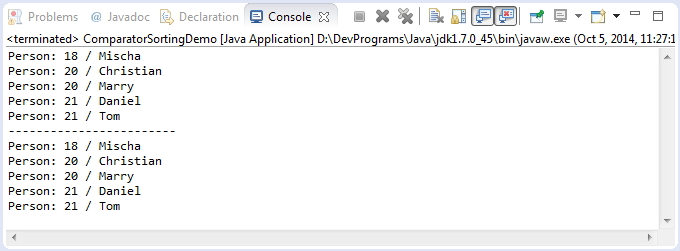#### View more Tutorials:

Maybe you are interested

These are online courses outside the o7planning website that we introduced, which may include free or discounted courses.

•Complete Step By Step Java For Testers
•*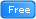* Java Database Connection: JDBC and MySQL
•Java JDBC with Oracle: Build a CRUD Application
•Java Object-Oriented Programming : Build a Quiz Application
•Understanding JDBC with PostgreSQL (A step by step guide)